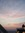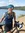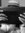Random Ramblings discussion

29 views
Random :D :P :) XD > Smiley Face

« previous 1

message 1: by Becky, AwesomeOne (new)

In this topic put a smiley face on how you are feeling!

message 2: by Becky, AwesomeOne (new)

message 3: by Sarah, The Random Nerd (new)

;]

(a bit tired but happy)

message 4: by (new)

message 5: by Sarah, The Random Nerd (new)

8)

message 6: by Becky, AwesomeOne (new)

:D Over the moon!

message 7: by (new)

message 8: by Becky, AwesomeOne (new)

Probably like this
Tired but happy at the same time
It looks funny!

(
D
(

message 9: by Becky, AwesomeOne (new)

The smiley face isn't supposed to be aligned with the eyes but theres nothing you can do

message 10: by (new)

message 11: by (new)

message 12: by Sarah, The Random Nerd (new)

message 14: by Sarah, The Random Nerd (new)

message 15: by Becky, AwesomeOne (new)

message 16: by (new)

message 17: by (new)

message 18: by Becky, AwesomeOne (new)

message 19: by Sarah, The Random Nerd (new)

:[[ I'M HUNGRY!

message 20: by Becky, AwesomeOne (new)

I am tired -.-

message 21: by (new)

message 22: by Becky, AwesomeOne (new)

YIKES!!! I could not sleep in to then!

message 23: by (new)=)))))))))))))))))))))

VERY Happy
I don't have school today!!!!!

message 24: by Sarah, The Random Nerd (new)

Yay Emily!

:)))))))))))))))))))))))))))))))))))))))))))))))))))))))))))))))))))))))))))))))))))))))))))))))))))))))))))))))))))))))))))))))))))))))))))))))))))))))))))))))))))))))))))))))))))))))))))))))))))))))))))))))))))))))))))))))))))))))))))))))))))))))))))))))))))))))))))))))))))))))))))

(Clearly, I'm miserable... I mean, what else could that mean...? ;) )

message 26: by Sarah, The Random Nerd (new)

Sorry, Emily! At least it's Friday, though. :)

message 27: by (last edited Sep 29, 2011 08:37PM) (new)I know.I won't be that bad.Except tommrow is the 3rd annv.of my grandparents dog dieing.She was two and attacked by a dog.She was so cute.I miss her.

=(

message 28: by Sarah, The Random Nerd (new)

Oh no!!! I'm so sorry!!! It's horrible to lose a pet. :( *hugs through the Internet*

message 29: by (new)Thanks for the hug.It really is awful.She was a Maltese and so adorable.I really want a dog for my grandparents or our family,but there still sad over Muffin(the dog)
Feb.11th 2006-Sept.30th 2008

I still remember crying in the Mutli Purpose Room the next day.I was 12.

message 30: by Becky, AwesomeOne (new)

Emily wrote: "I know.I won't be that bad.Except tommrow is the 3rd annv.of my grandparents dog dieing.She was two and attacked by a dog.She was so cute.I miss her.

=("

That is so sad! I know how it feels to lose a pet

message 31: by (new)Becky wrote: "Emily wrote: "I know.I won't be that bad.Except tommrow is the 3rd annv.of my grandparents dog dieing.She was two and attacked by a dog.She was so cute.I miss her.

=("

That is so sad! I know how ..."

I also lost a bird recently.Which is awful.

message 32: by Sarah, The Random Nerd (new)

Aww, Emily... You're having such a rough time lately. :( I'm sorry you had a bad day. I hope everything gets better for you. <3

message 33: by (new)Aw, I'm sorry Emily. We're here if you ever need someone to talk too.

message 34: by (new)

message 36: by (new)

message 37: by (new)

message 38: by (new)

message 39: by (new)

message 40: by (new)

message 41: by (new)what I'm wishing for right now

----
--
[]
--
----

that was supposed to be a tardis.

message 42: by (new)

message 43: by Sarah, The Random Nerd (new)

It's Friday now...SD is tired.

message 44: by (new)

message 45: by (new)Robin wrote: "Robin is tired, too. But Robin is always tired. XD"

So is Frances.

message 48: by Sarah, The Random Nerd (new)

Uh oh. Mischievous Khali, eh? BRING IT ON. *takes out stupid argument hat*

message 50: by Sarah, The Random Nerd (new)

« previous 1